# Samacheer Kalvi 11th Economics Solutions Chapter 2 Consumption Analysis

Guys who are planning to learn the fundamentals of Economics Solutions can avail the handy study material Samacheer Kalvi Class 11th Economics Solutions Chapter 2 Consumption Analysis Questions and Answers here. Refer to the Samacheer Kalvi Economics Solutions for Class 11th PDF available and score better grades. Simply click on the quick links available for Samacheer Kalvi 11th Economics Solutions Chapter 2 Consumption Analysis and prepare all the concepts in it effectively. Take your preparation to the next level by availing the Tamilnadu State Board Solutions for Class 11th Economics Solutions Chapter 2 Consumption Analysis prepared by subject experts.

## Tamilnadu Samacheer Kalvi 11th Economics Solutions Chapter 2 Consumption Analysis

Do you feel the concept of Economics Solutions difficult to understand? Not anymore with our Samacheer Kalvi for Class 11th Economics Solutions Chapter 2 Consumption Analysis Questions and Answers. All the Solutions are given to you with detailed explanation and you can enhance your subject knowledge. Use the Samacheer Kalvi Textbook Chapter 2 Consumption Analysis Solutions PDF free of cost and prepare whenever you want.

### Samacheer Kalvi 11th Economics Consumption Analysis Text Book Back Questions and Answers

Part – A

Multiple Choice Questions

11th Economics Chapter 2 Book Back Answers Question 1.
Pick the odd one out
(a) Luxuries
(b) Comforts
(c) Necessaries
(d) Agricultural
(d) Agricultural

Samacheer Kalvi 11th Economics Solution Chapter 2 Question 2.
Choice is always constrained or limited by the …………………… of our resources.
(a) Scarcity
(b) Supply
(c) Demand
(d) Abundance
(a) Scarcity

11th Economics Chapter 2 Question 3.
The chief exponent of the Cardinal utility approach was
(a) J.R.Hicks
(b) R.G.D.Allen
(c) Marshall
(d) Stigler
(c) Marshall

Samacheer Kalvi Guru 11th Economics Question 4.
Marginal Utility is measured by using the formula of ……………………..
(a) TUn – TUn-1
(b) TUn – TUn+1
(c) TUn + TUn+1
(d) TUn – TUn+1
(a) TUn – TUn-1

Samacheer Kalvi Guru 11 Economics Question 5.
When marginal utility reaches zero, the total utility will be
(a) Minimum
(b) Maximum
(c) Zero
(d) Negative
(b) Maximum

11th Economics Samacheer Kalvi Question 6.
Gossen’s first law is known as ……………………..
(a) Law of equi-marginal utility
(b) Law of diminishing marginal utility
(c) Law of demand
(d) Law of Diminishing returns.
(b) Law of diminishing marginal utility

Samacheer Kalvi 11th Economics Question 7.
The basis for the law of demand is related to
(a) Law of diminishing marginal utility
(b) Law of supply
(c) Law of Equi-marginal utility.
(d) Gossen’s Law.
(a) Law of diminishing marginal utility

11th Economics Solutions Samacheer Kalvi Question 8.
The concept of consumer’s surplus is associated with ……………………….
(b) Marshall
(c) Robbins
(d) Ricardo
(b) Marshall

Samacheer Kalvi 11th Economics Solutions Question 9.
Given the potential price is Rs.250 and the actual price is Rs.200. Find the consumer surplus,
(a) 375
(b) 175
(c) 200
(d) 50
(d) 50

Indifference curve approach is based on ………………………..
(a) Ordinal approach
(b) Cardinal approach
(c) Subjective approach
(d) Psychological approach
(a) Ordinal approach

Samacheer Kalvi Class 11 Economics Solutions Question 11.
The concept of elasticity of demand was introduced by
(a) Ferguson
(b) Keynes
(d) Marshall
(d) Marshall

Economics Chapter 2 Question 12.
Increase in demand is caused by ……………………..
(a) Increase in tax
(b) Higher subsidy
(c) Increase in interest rate
(d) decline in population
(b) Higher subsidy

11 Economics Samacheer Kalvi Question 13.
The movement on or along the given demand curve is known as _______.
(a) Extension and contraction of demand.
(b) Shifts in the demand,
(c) Increase and decrease in demand.
(d) All the above
(a) Extension and contraction of demand.

Samacheer Kalvi 11th Economics Book Question 14.
In case of relatively more elastic demand, the shape of the curve is ……………………..
(a) Horizontal
(b) Vertical
(c) Steeper
(d) Flatter
(d) Flatter

Economics Class 11 Samacheer Kalvi Question 15.
A consumer is in equilibrium when marginal utilities from two goods are
(a) Minimum
(b) Inverse
(c) Equal
(d) Increasing
(c) Equal

Chapter 2 Of Economics Class 11 Question 16.
Indifference curve was first introduced by ………………………..
(a) Hicks
(b) Allen
(c) Keynes
(d) Edgeworth
(d) Edgeworth

Samacheer Kalvi Economics Question 17.
The elasticity of demand is equal to one indicates
(a) Unitary Elastic Demand
(b) Perfectly Elastic Demand
(c) Perfectly Inelastic Demand
(d) Relatively Elastic Demand
(a) Unitary Elastic Demand

Samacheer Kalvi Economics 11th Question 18.
The locus of the points which gives the same level of satisfaction is associated with ……………………….
(a) Indifference Curves
(b) Cardinal Analysis
(c) Law of Demand
(d) Law of Supply
(a) Indifference Curves

Economics Chapter 2 Class 11 Question 19.
Ordinal Utility can be measured by
(a) Ranking
(b) Numbering
(c) Wording
(d) None of these
(a) Ranking

Economics Class 11 Chapter 2 Question 20.
The indifference curve are ………………………..
(a) Vertical
(b) Horizontal
(c) Positive sloped
(d) Negatively sloped
(d) Negatively sloped

Part – B

Answer the following questions in one or two sentences

11th Economics Book Answers Question 21.
Define Utility.
Utility is the capacity of a commodity to satisfy human wants.

Class 11 Economics Chapter 2 Question 22.
Mention the classifications of wants.
(a) Necessaries
(b) Comforts
(c) Luxuries.

Question 23.
There are two basic approaches, namely:
1. Utility approach

• The utility approach involves the use of measurable (cardinal) utility to study consumer behaviour.
• Marshall is the chief exponent of the utility approach to the theory of demand. It is known cardinal utility analysis or Marginal utility analysis or Marshallian utility analysis.

2. Indifference curve approach

• The indifference curve approach was the idea of comparable utility [ordinal utility] J.R. Hicks and R.G.D. Allen introduced the indifference curve approach.

Question 24.
What are the degrees of price elasticity of Demand?
The Degrees of Price Elasticity of Demand:

1. Perfectly Elastic Demand (Ep = α)
2. Perfectly Inelastic Demand (Ep = 0)
3. Relatively Elastic Demand (Ep >1)
4. Relatively Inelastic Demand (Ep < 1)
5. Unitary Elastic Demand (Ep =1).

Question 25.
State the meaning of indifference curves?

1. The Consumer is rational and his aim is to derive maximum satisfaction.
2. The utility cannot be cardinally measured but can be ranked or compared or ordered by an ordinal number such as I, II, III, and so on.
3. The indifference curve approach is based on the concept “Diminishing Marginal Rate of Substitution”.
4. The consumer is consistent. This assumption is called the assumption of transitivity.

Question 26.
Write the formula of consumer surplus.
Consumer’s surplus = Potential price – Actual price.
Consumer’s surplus = TU-(P × Q)
TU – Total Utility,
P – Price,
Q – Quantity.

Question 27.
What are Giffen goods? Why it is called that?
Giffen Paradox: The Giffen good or inferior good is an exception to the law of demand. When the price of inferior goods, falls, the poor will buy less and vice versa.

Part – C

Answer the following questions in One Paragraph

Question 28.
Describe the feature of human wants.
The Feature of Human:

1. Wants are unlimited
2. Wants become habits
3. Wants are satiable
4. Wants are alternative
5. Wants are competitive
6. Wants are complementary
7. Wants are recurring.

Question 29.
Mention the relationship between marginal utility and total utility.
Total Utility:

1. If Total utility increases
2. If Total utility reaches maximum.
3. If Total utility diminishes

Marginal Utility:

1. Marginal utility declines
2. Marginal utility reaches zero
3. Marginal utility becomes negative

MUn = TUn – TUn-1.

Question 30.
Explain the concept of consumer’s equilibrium with a diagram.
Consumer’s surplus is the difference between the potential price and actual price. Consumer’s surplus = Potential price – Actual price.
(or)
Consumer’s surplus = TU – (P × Q)
TU – Total Utility, P – Price, Q – Quantity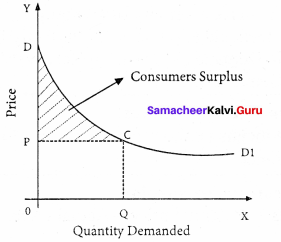In the diagram X – axis shows the amount demanded and Y – axis represents the price. DD shows the consumer’s utility from the purchase of different amounts of commodity.
Hence Actual price OPCQ
Potential price ODCQ
Consumer’ surplus = ODCQ – OPCQ
= PCD.

Question 31.
Explain the theory of “ Consumer’s Surplus ”?
Alfred Marshall defines consumer’s surplus as “the excess of price which a person would be willing to pay a thing rather than go without the thing, over that which he actually does pay is the economic measure of this surplus satisfaction. This may be called consumer’s surplus”.

Question 32.
Distinguish between extension and contraction of demand.
If the changes in the quantity demanded is due to the change in price alone then it is called extension and contraction of demand. Buying more at lower price is extension of demand and less at higher price is contraction of demand.

Question 33.
What are the properties of indifference curves?

1. Indifference curve must have negative slope.
2. Indifference curves are convex to the origin.
3. Indifference curve cannot intersect.
4. Indifference curves do not touch the horizontal or vertical axis.

Question 34.
Briefly explain the concept of consumer’s equilibrium.
Consumer’s equilibrium refers to a situation under which a consumer spends his entire income on purchase of a goods, in such a manner that it gives him maximum satisfaction.
The consumer reaches equilibrium at the point where the budget line is tangent on the indifference curve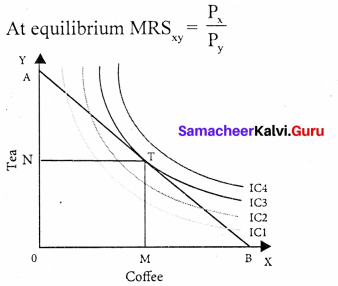T is the point of Equilibrium as budget line AB is tangent on indifference curve IC3, the upper IC implies maximum level of satisfaction.

Part – D

Question 35.
Explain the law of demand and its exceptions.
The law of demand was first stated by Augustin Cournot in 1838. Later it was refined and elaborated by Alfred Marshall.
Definition:
The law of demand says as “The quantity demanded increases with a fall in price and diminishes with a rise in price” -Marshall.

Assumptions of the law:

1. The income, taste, habit, and preference of the consumer remain the same.
2. No change in the prices of related goods.
3. No substitutes for the commodity.
4. The demand for the commodity must be continuous.
5. No change in the quality of the commodity.

If there is change even in one of these assumptions, the law will not operate.
Demand schedule: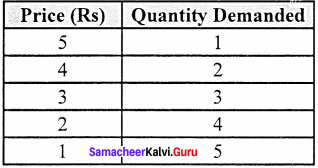From the above schedule if the price of the good is 5 then the quantity demanded is 1 unit and if the price decrease to 1 quantity demanded raises to 5 which shows the inverse relationship between price and quantity demanded.

Diagram: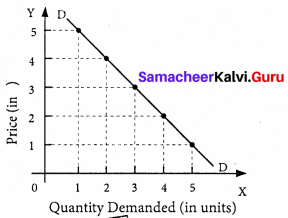In the above diagram, X axis represents the quantity demanded and Y axis represents the price. DD is the demand curve which has a negative slope. It indicates that when price falls, the demand expands and when price rises, the demand contracts.

Market demand for a commodity: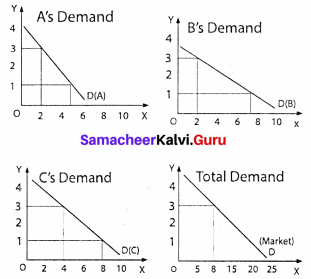The market demand curve for a commodity is derived by adding the quantum demanded of the commodity by all the individuals in the market.
Exceptions to the law of demand:

1. There are some unusual demand curves which slopes upwards from left to right. It is known as exceptional demand curve.
2. In the case of exceptional demand curve a fall in price brings about contraction and a rise in price brings about extension of demand.

Reasons for exceptional demand curve:

2. Veblen or demonstration
3. Ignorance
4. Speculative effect
5. Fear of shortage

Question 36.
Elucidate the law of diminishing marginal utility with diagram.
H.H. Gosen, an Austrain Economist first formulated this law in 1854. Hence Jevons called this law as “Gossen’s first law of consumption”. Marshall perfected this law on the basis of cardinal analysis and it is based on the characteristics of human wants, it wants are satiable.

Definition:
Marshall states that “The additional benefit which a person derives from a given increase of his stock of a thing diminishes with every increase in the stock that he already has”
Assumptions:

1. Utility can be measured cardinally.
2. The marginal utility of money remains constant.
3. Consumer is a rational economic man.
4. The units of the commodity consumed must be reasonable in size.
5. The commodity consumed should be homogenous in all aspects.
6. Consumption takes place continuously at a given period of time.
7. No change in the taste, habits, preferences, fashions, income and character of the consumer during the process of consumption.

Explanation:
The law states that if a consumer continues to consume more of more units of the same commodity, its marginal utility diminishes.

Illustration:
This law can be explained with a simple illustration. Suppose a consumer wants to consume apples one after another the utility from the first apple is 20. But the utility from the second apple will be less than the first (say 15), the third less than the second (say 10) and so on. Finally, the utility from the fifth apple becomes zero.

The utilities from sixth and seventh apples are negative. This tendency is called “The . law of diminishing marginal utility’”.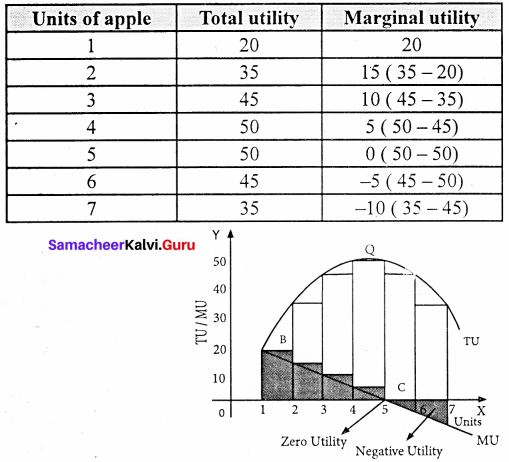From the above table and diagram. We find that the total utility goes on increasing but at a diminishing rate. Whereas marginal utility goes on diminishing. When marginal utility becomes zero, the total utility is maximum, when marginal utility becomes negative, the total utility diminishes.

Criticisms:

1. As utility is subjective,it cannot be measured numerically.
2. This law is based on the unrealistic assumptions.
3. This law is not applicable to indivisible commodities.

Question 37.
Explain the law of Equi-marginal utility.
To satisfy unlimited wants a consumer need more than one commodity. So, the law of diminishing marginal utility is extended and is called “Law of equi-marginal utility”. It is also called the “Law of substitution” “The law of consumer’s equilibrium”, “Gossen second law” and “The law of maximum satisfaction”.

Definitio :
Marshall states the law as, “If a person has a thing which he can put to several uses, he will distribute it among these uses in such a way that it has the same marginal utility in all. For if it had a greater marginal utility in one use than another he would gain by taking away some of it from the second use and applying it to first”.
Assumptions:

1. The rational consumer wants to maximize his satisfaction.
2. Utility is measurable cardinally.
3. The marginal utility of money remains constant.
4. The income of the consumer is given.
5. There is perfect competition in the market.
6. The prices of the commodities are given.
7. The law of diminishing marginal utility operates.

Explanation:
The law can be explained with the help of an example. Suppose a consumer wants to spend his limited income on Apple and Orange. He is said to be in equilibrium, only when he gets maximum satisfaction with his limited income. Therefore, he will be in equilibrium, when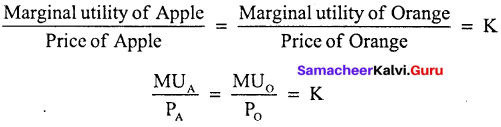K – Constant marginal unity of money

Table: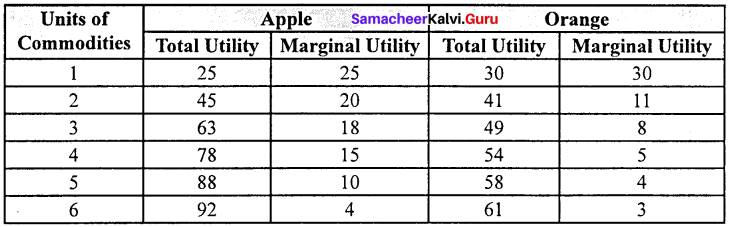Let us assume that the consumer wants to spend his entire income (Rs.11) on Apple add Orange. The price of an Apple and Orange is Rs. 1 each.
If the consumer wants to attain maximum utility he should buy 6 units of Apples and 5 units or Oranges so that he can get 150 units.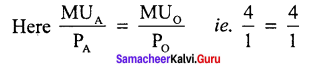Diagram: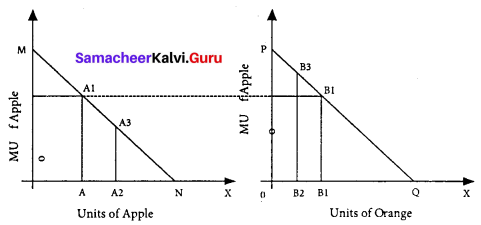In the diagram X – axis represent amount of money spent and Y – axis marginal utilities of Apple and Orange. If the consumer spends Rs. 6 on Apple and Rs. 5 on Orange, the marginal utilities of both are equal (ie) AA, = BB,. Hence he gets maximum utility.

Question 38.
What are the methods of measuring Elasticity of demand?
There are three methods of measuring elasticity of demand.

1. The percentage method: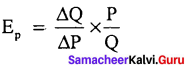It is also known as ratio method, when we measure the ratio as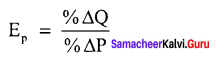% ∆Q = perCentage change in demand, %∆P = Percentage change in price.

2. Total outlay method:
Marshall suggested that the simplest way to decide whether demand is elastic or inelastic is to examine the change in total outlay of the consumer or total revenue of the firm.
Total revenue = Price × Quantity sold
TR = P × Q
Total outlay method: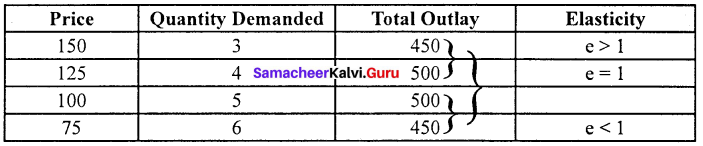Demand is elastic, if there is inverse relation between price and total outlay and direct relation means inelastic. Elasticity is unity when total outlay is constant.

Question 3.
Point or geometrical elasticity:
The point elasticity of a linear demand curve is shown by the ratio of the segments of the line to the right and to the left of the particular point.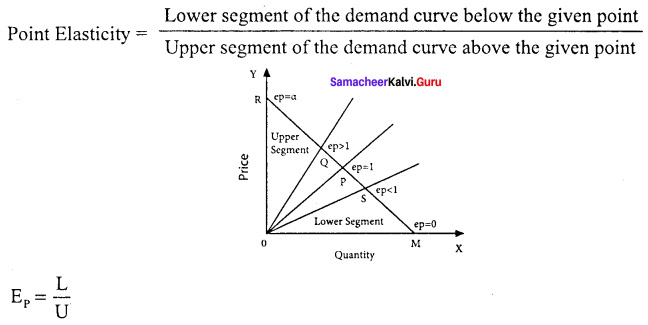EP – Point Elasticity, L – Lower Segment,U – Upper Segment.

Part – A

Choose the best options

Question 1.
The utility cannot be measured, because the utility is ………………………. concept.
(a) Social
(b) Subjective
(c) Political
(d) Scientific
(b) Subjective

Question 2.
_________ law is helpful in attaining social justice.
(a) Law of equi-margional utility
(b) Law of demand
(c) Law of diminishing marginal utility
(d) Law of marginal utility
(c) Law of diminishing marginal utility

Question 3.
Consumer surplus is …………………….
(a) Potential price – Actual price
(b) MV = TV – TV
(c) Demand = Supply
(d) None
(a) Potential price – Actual price

Question 4.
Income elasticity of demand is degree of responsiveness of change in demand to
(a) Change is price
(b) Elasticity of demand
(c) Change in substitutes
(d) Change in income
(d) Change in income

Question 5.
Marshallian utility approach is …………………….. analysis.
(a) Subjective
(b) Psychological
(c) Ordinal
(d) Cardinal
(d) Cardinal

Question 6.
_________ is the major determinant of demand.
(a) Consumption
(b) Price
(c) Supply
(d) All the above
(b) Price

Question 7.
Principle of Economics” was defined by ………………….
(a) Marshall
(b) Hicks
(c) Allen
(d) Keynes
(a) Marshall

Question 8.
The formula for point method
(a) Upper segment / Lower segment
(b) Middle segment / Upper segment
(c) Lower segment / Upper segment
(d) Upper segment / Left segment
(c) Lower segment / Upper segment

Question 9.
What is the other name of the budget line?
(a) Price ratio line
(b) Quantity ratio line
(c) Equilibrium ratio line
(d) Compulsory ratio line
(a) Price ratio line

Question 10.
_________ is the basis of all the laws of consumption.
(a) Law of demand
(b) Law of consumerism
(c) Law of elasticity
(d) Law of diminishing marginal utility
(d) Law of diminishing marginal utility

Question 11.
Give the consumer’s surplus is 75 and the actual price 372, Find the potential price
(a) 447
(b) 50
(c) 375
(d) 474
(a) 447

Choose the correct statement

Question 1.
(a) Consumption is the beginning of economic science
(b) Production is the beginning of economic science
(c) Distribution is the beginning of economic science
(d) Exchange is the beginning of economic science
(a) Consumption is the beginning of economic science

Question 2.
(a) The law of diminishing marginal utility is called as Gresham’s law.
(b) The law Equi marginal utility is called as Marshall’s law.
(c) The law of diminishing marginal utility is called as Gossen’s first law of consumption.
(d) The law of demand is called as Gossen’s second law of consumption.
(c) The law of diminishing marginal utility is called as Gossen’s first law of consumption.

Match the following and choose the answer using the codes given below

Question 3.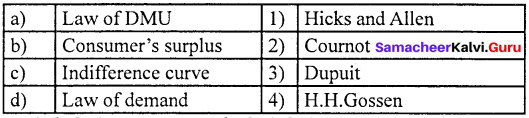(a) 1 2 3 4
(b) 3 4 2 1
(c) 2 3 4 1
(d) 4 3 2 1
(b) 3 4 2 1

Question 4.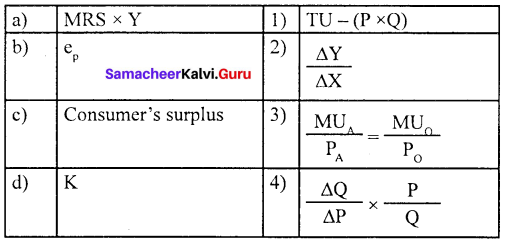(a) 4 3 2 1
(b) 2 1 4 3
(c) 3 4 2 1
(d) 3 1 4 2
(d) 3 1 4 2

Choose the odd one out

Question 5.
(b) Demonstration effect
(c) Speculative effect
(d) Edge worth approach
(d) Edge worth approach

Question 6.
(a) F.W. Edge worth
(b) Alfred Marshall
(c) Vilfredo Pareto
(d) J.R. Hicks and R.G.D. Allen
(b) Alfred Marshall

Question 7.
(a) Percentage method
(b) Point method
(c) Total outlay method
(d) Income elasticity method
(d) Income elasticity method

Choose the incorrect pair

Question 8.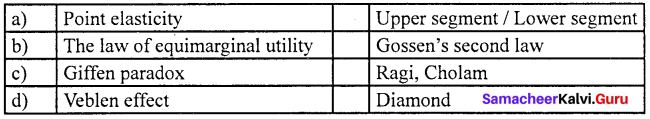(a) Point (i) Upper segment/Lower segment.

Question 9.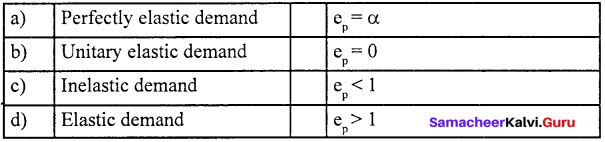(b) Unptary elastic demand (i) ep= 0.

Question 10.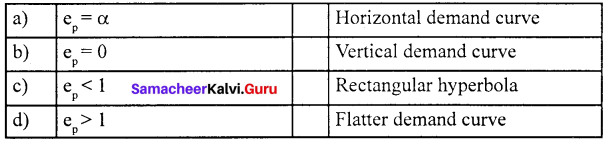(c) ep < 1 (iii) Rectangular hyperbola

Analyze the reason for the following

Question 11.
Assertion (A): Savings and demand are inversely related.
Reason (R): Increased savings leads to a decrease in consumption.
(a) Both (A) and (R) are true, (R) is the correct explanation of (A)
(b) Both (A) and (R) are true, (R) is not the correct explanation of (A)
(c) (A) is true but, (R) is false.
(d) Both (A) and (R) are false.
(a) Both (A) and (R) are true, (R) is the correct explanation of (A)

Question 12.
Assertion (A) : When the price of an inferior goods fall, the poor will buy less.
Reason (R) : Poor consume less amount of inferior goods.
(a) Both (A) and (R) are true
(b) Both (A) and (R) are false
(c) (A) is true but (R) is false.
(d) (A) is false but (R) is true.
(c) (A) is true but (R) is false.

Choose the incorrect statement

Question 13.
(a) The exceptional demand curve slopes upwards,
(b) The good salt is price inelastic
(c) Elasticity is unity when total outlay is constant
(d) Demand is more elastic in the short run than is long run
(a) The exceptional demand curve slopes upwards

Question 14.
(a) The law of DMU is based on the characteristics of human wants.
(b) The law of consumer surplus is based on the law of DMU
(c) The indifference curve approach is based on scale of preference.
(d) None of the above.
(d) None of the above.

Fill in the blanks with the suitable option given below

Question 15.
Diamond – water paradox was given by
(b) Marshall
(c) Robbins
(d) Samuelson

Question 16.
_________ law is helpful in attaining social justice.
(a) Law of demand
(b) Law of diminishing marginal utility
(c) Law of equi-marginal utility
(d) None of the above
(b) Law of diminishing marginal utility

Question 17.
_________ is the basis of all the laws of consumption.
(a) Law of demand
(b) Law of consumerism
(c) Law of elasticity
(d) Law of diminishing marginal utility
(d) Law of diminishing marginal utility

Choose the best option

Question 18.
_________ is the major determinant of demand.
(a) Consumption
(b) Price
(c) Supply
(d) All the above
(b) Price

Question 19.
If the total utility is maximum then marginal utility is _________
(a) Zero
(b) Negative
(c) Positive
(d) Maximum
(a) Zero

Question 20.
Mathematically consumer’s surplus is
(a) TU – TUn-1
(b) TR – (P×Q)
(c) TU – (P×Q)
(d) TC – (Q×P)
(c) TU-(P×Q)

Part – B

Answer the following questions in one or two sentences

Question 1.
Define “Consumption”?
Consumption plays an important role in Economics. “ Consumption is the sole end and object of economic activity” – J.M. Keynes. Consumption is the beginning of economic science. In the absence of consumption, there can be no production; exchange, or distribution. Consumption is also an end of production. Producers produce goods to satisfy the wants of the people.

Question 2.
What is the law of demand?
“The quantity demanded increases with a fall in price and diminishes with a rise in price” – Marshall.

Question 3.
Write the characteristics of demand?
Characteristics of demand:

1. Price: Demand is always related to price.
2. Time: Demand always means demand per unit of time, per day, per week, per month on per year.
3. Market: Demand is always related to the market, buyer and sellers.
4. Amount: Demand is always a specific quantity that a consumer is willing to purchase.

Question 4.
What is marginal utility?
Marginal utility is the utility derived from the last or marginal unit of consumption.

Question 5.
What is demand ?
Demand is the desire backed by the ability to pay and the willingness to buy it.

Question 6.
What is elasticity of demand?
Elasticity of demand is the degree of responsiveness of the quantity demand for a commodity to a change in its price.

Question 7.
What are the determinants of elasticity of demand?

1. Availability of substitutes
2. Proportion of consumer’s income
3. Number of uses of commodity
4. Complementarity between goods.

Part – C

Answer the following questions in one Paragraph

Question 1.
What is an indifference map?
An indifference map is a family or collection or set of indifference curves corresponding to different levels of satisfaction. In the diagram, the indifference curves IC1, IC2 and IC3 represent the indifference map, upper IC representing a higher level of satisfaction compared to lower IC.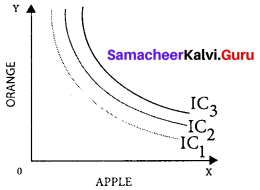Question 2.
Write the importance of the law of diminishing marginal utility?

1. This law of DMU is one of the fundamental laws of consumption. It has applications in several fields of study.
2. The law of DMU is the basis for other consumption laws such as the law of Demand, Elasticity of Demand, Consumer Surplus, and the Law of Substitution, etc.
3. The law emphasizes the equitable distribution of wealth. The MU of money to the more -moneyed is low. Hence redistribution of income from rich to poor is justified.

Question 3.
What are the determinants of demand?

1. Changes in tastes and fashions
2. Change in weather
3. Taxation and subsidy
4. Change in expections
5. Changes in savings